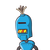# Q9. Determine the integer, a) Whose product with (-7) is 154 b) When it is divided by ( -9), the answer is 23.

Q9. Determine the integer,
a) Whose product with (-7) is 154
b) When it is divided by ( -9), the answer is 23.

### 1 thought on “Q9. Determine the integer,<br /> a) Whose product with (-7) is 154<br /> b) When it is divided by ( -9), the answer is 23.”

1.()ʜs ʀ ɪʜ (7) ɪs 154.

ɴs=(22)

(ʙ)ʜɴ ɪ ɪs ɪɪ ʙʏ (9), ʜ ɴsʀ ɪs 23.

ɴs=(207)